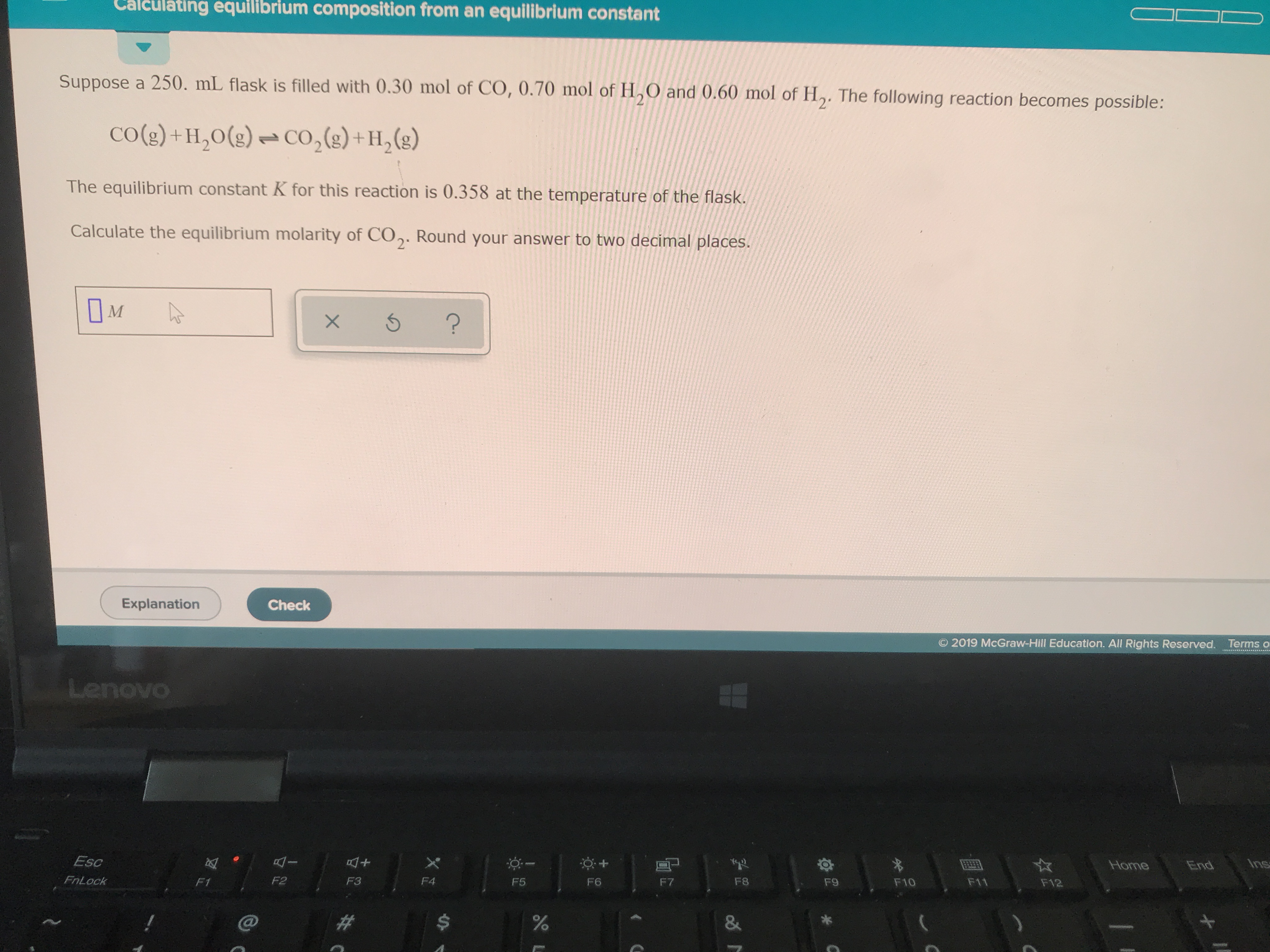# Suppose a 250 mL flask is filled with 0.30 mol of CO, 0.70 mol of H2O and 0.60 mol of H2. The following reaction becomes possible: CO(g) + H2O(g) <-> CO2(g) + H2(g). The equilibrium constant K for this reaction is 0.358 at the temperature of the flask. Calculate the equilibrium molarity of CO2.